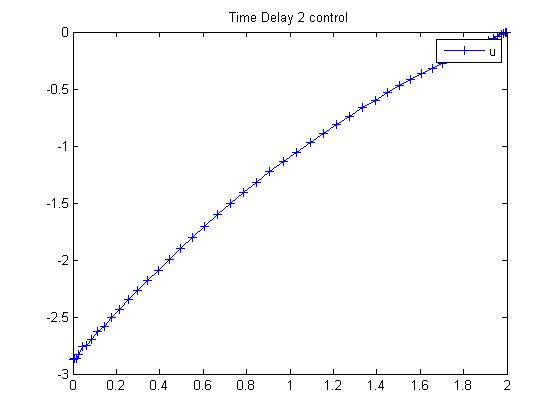115  Time Delay 2

ITERATIVE DYNAMIC PROGRAMMING, REIN LUUS

8.3.2 Example 2

CHAPMAN & HALL/CRC Monographs and Surveys in Pure and Applied Mathematics

Linear time-delay system considered by Palanisamy et al.

115.1  Problem Formulation

Find u over t in [0; 2 ] to minimize

 J = x2(tF)

subject to:

 dx1 dt
= t*x1 + x1(ttau) + u
 dx2 dt
= x12 + u2
 tau = 1

The initial condition are:

 x(t<=0) = [1  0]
 −inf <= u <= inf

Reference: 

115.2  Problem setup

toms t
p1 = tomPhase('p1', t, 0, 2, 50);
setPhase(p1);

tomStates x1 x2
tomControls u

% Initial guess
x0 = {icollocate({x1 == 1; x2 == 0})
collocate(u == 0)};

% Boundary constraints
cbnd = initial({x1 == 1; x2 == 0});

% Expression for x1(t-tau)
tau = 1;
x1delayed = ifThenElse(t<tau, 1, subs(x1,t,t-tau));

% ODEs and path constraints
ceq = collocate({
dot(x1) == t.*x1 + x1delayed + u
dot(x2) == x1.^2 + u.^2});

% Objective
objective = final(x2);

115.3  Solve the problem

options = struct;
options.name = 'Time Delay 2';
solution = ezsolve(objective, {cbnd, ceq}, x0, options);
t  = subs(collocate(t),solution);
u  = subs(collocate(u),solution);
Problem type appears to be: lpcon
Starting numeric solver
===== * * * =================================================================== * * *
TOMLAB - Tomlab Optimization Inc. Development license  999001. Valid to 2011-02-05
=====================================================================================
Problem: ---  1: Time Delay 2                   f_k       4.796108536142883200
sum(|constr|)      0.000000305572156922
f(x_k) + sum(|constr|)      4.796108841715040100
f(x_0)      0.000000000000000000

Solver: snopt.  EXIT=0.  INFORM=1.
SNOPT 7.2-5 NLP code
Optimality conditions satisfied

FuncEv    1 ConstrEv   32 ConJacEv   32 Iter   27 MinorIter  137
CPU time: 0.171875 sec. Elapsed time: 0.204000 sec.

115.4  Plot result

figure(1)
plot(t,u,'+-');
legend('u');
title('Time Delay 2 control');